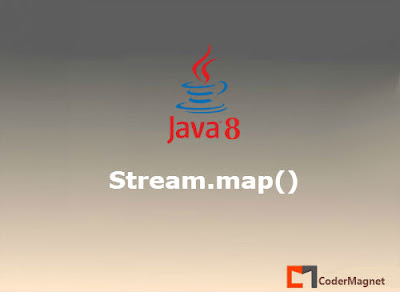•## Monday, 30 May 2016

### The Stream.map() function in Java 8## Stream.map() method in Java 8

The Stream.map(Function) methods returns a stream consisting of the results of applying the given function to the elements of this stream.

For Example:
If we have a Stream like this {10, 20 , 30 ,40 ,50}.
Then applying a map function like this {10, 20 , 30 ,40 ,50}.map( x -> x=x+5 )
will return a Stream like {15, 25, 35, 45, 55}.

Lets consider the following examples.

#### Program 1: A Simple One

``````
package codermag.net.java8;

import java.util.Arrays;
import java.util.List;
import java.util.stream.Stream;

public class StreamMapExample {
public static void main(String[] args) {

List<Integer> myList = Arrays.asList(10,20,30,40,50);

//Convert this list to a stream
Stream<Integer> items=myList.stream();

Stream<Integer> newStream=items.map(x -> x=x+5);

newStream.forEach(System.out :: println);

}
}

``````

Output:
``````
15
25
35
45
55
``````

#### Program 2: Some simple String operations.

``````
package codermag.net.java8;

import java.util.Arrays;
import java.util.List;
import java.util.function.Supplier;
import java.util.stream.Stream;

public class StreamMapExample {
public static void main(String[] args) {

List<String> myList = Arrays.asList("HelloWorld","Java","CoderMagnet");

//A Supplier is a supplier of stream. Each get() will give you a copy of the Stream
Supplier<Stream<String>> streamSupplier=() -> myList.stream();
Stream<String> stream1=streamSupplier.get();
Stream<String> stream2=streamSupplier.get();

Stream<String> newStream=stream1.map(x -> x.substring(2));
newStream.forEach(System.out :: println);

Stream<String> newStream2=stream2.map(String :: toUpperCase);
newStream2.forEach(System.out :: println);

}
}
``````

A stream can be used only once. We are using a supplier here only because we want to use the same stream of elements 2 times.
On the 1st copy of the Stream we apply a substring() function.
On the 2nd cop of the Stream we apply a toUpperCase() function.
The output looks like this.

Output:
``````
lloWorld
va
derMagnet
HELLOWORLD
JAVA
CODERMAGNET
``````

#### Program 3: A little more complex program, trust me its easy.

``````
package codermag.net.java8;

import java.util.Arrays;
import java.util.List;
import java.util.stream.Stream;

public class StreamMapExample {
public static void main(String[] args) {

List<String> myList = Arrays.asList("HelloWorld","Java","CoderMagnet");
Stream<String> stream1= myList.stream();

Stream<String> newStream=stream1.map(s ->
{
System.out.format("String---- %s %s\n",s,s.toUpperCase());
return s.toUpperCase();
});

//Running a loop will call the above "map" method over each element
newStream.forEach(x -> x=x);

}
}
``````

Here the mapping function has a function body.
Whenever we run forEach Loop over the elements, this method body will be called every time.
The output will look like this below.

Output:
``````
String---- HelloWorld HELLOWORLD
String---- Java JAVA
String---- CoderMagnet CODERMAGNET
``````